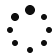The interactive flash videos below will show you how to do more complex things with SPSS, including comparing sets of data with multiple variables.

In this section we will start to consider more complicated analyses (specifically, the analysis of data when we have multiple independent variables). Here, you will find a series of interactive tutorials designed to guide you through these more complicated statistical tests using SPSS. (You do not need to be working on a computer with SPSS installed.)

#### 1. Two-Way Independent ANOVA

A two-way independent analysis of variance (ANOVA) is an extension of one-way independent ANOVA. In this case it is used to analyse the results from an entirely between-participants design with two independent variables (where participants are only assigned to one condition for each variable) and one dependent variable. This tutorial will interactively talk you though how to run this test in SPSS, and interpret the output produced.

2. Two-Way Repeated-Measures ANOVA

A two-way repeated measures analysis of variance (ANOVA) is used when you have two independent variables in a within-participants design experiment. This means that participants give data for all possible experimental conditions. This tutorial will interactively show you how to use SPSS to run this test, and interpret the output produced.

#### 3. Two-Way Mixed ANOVA

This tutorial will walk you through how to run and interpret a two-way mixed analysis of variance (ANOVA) in SPSS. You would use this test when you have one dependent variable, and two independent variables: one which is within-participants and another which is between-participants.

#### 4. Entering Factorial Data

When collecting data from an experiment with a factorial design (i.e. an experiment with more than one independent variable), you need to make sure that you enter your data into SPSS correctly… otherwise you will not be able to carry out a meaningful analysis. The way you enter data will depend on your experimental design. This tutorial will walk you through the different ways of entering data for the different factorial designs.

#### 5. Multiple Regression

Multiple regression is an extension of simple linear regression. It allows you to investigate the relationship between multiple variables simultaneously… but more than that, it allows you to model this relationship, enabling you to make predictions about what one variable will do based on the scores of some other variables. This tutorial will show you how to carry out and interpret a multiple regression analysis in SPSS.

#### 6. Multiple Regression Assumptions

When running a Multiple Regression, there are several assumptions that your data need to meet in order for your analysis to be reliable and valid. This tutorial will talk you though these assumptions and how they can be tested using SPSS.

#### 7. Factor Analysis

Factor analysis allows you to look at the relationships between a large number of variables (for example, questions on a questionnaire), to see whether they can be grouped and reduced into a smaller, more meaningful set of factors. This can help you to better understand the variance in your data. This tutorial will show you how to run and interpret a Factor Analysis using SPSS.